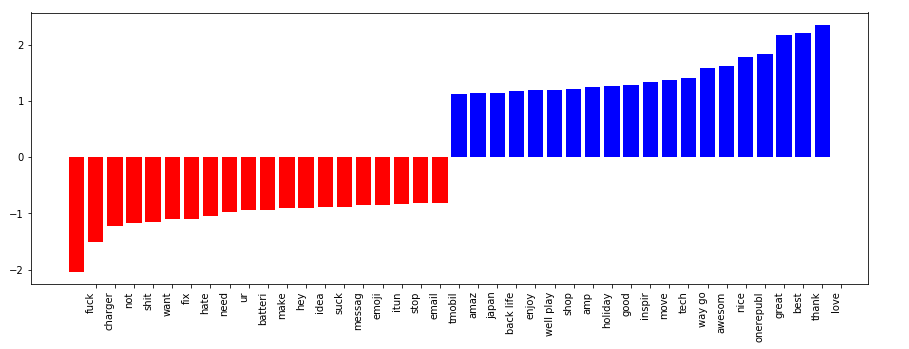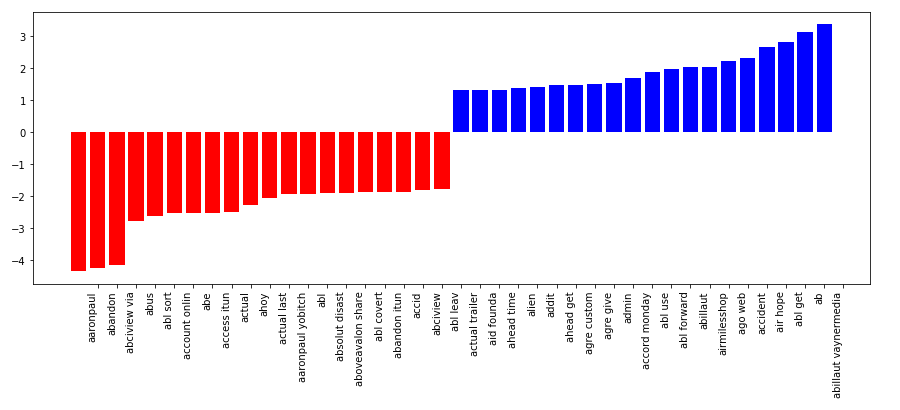# #StackBounty: #python #scikit-learn #pipeline #tf-idf #feature-selection Sentiment analysis Pipeline, problem getting the correct featu…

### Bounty: 50

In the following example I use a twitter dataset to perform sentiment analysis. I use sklearn pipeline to perform a sequence of transformations, add features and add a classifer. The final step is to visualise the words that have the higher predictive power. It works fine when I don’t use feature selection. However, when I do use it the results that I get make no sense. I suspect that when feature selection is applied the order of the text features changes. Is there a way to work around that?

``````from sklearn.base import BaseEstimator, TransformerMixin
from sklearn.pipeline import Pipeline, FeatureUnion

features= [c for c in df.columns.values if c  not in ['target']]
target = 'target'

#train test split
X_train, X_test, y_train, y_test = train_test_split(df[features], df[target], test_size=0.2,stratify = df5[target], random_state=0)

#Create classes which allow to select specific columns from the dataframe

class NumberSelector(BaseEstimator, TransformerMixin):

def __init__(self, key):
self.key = key

def fit(self, X, y=None):
return self

def transform(self, X):
return X[[self.key]]

class TextSelector(BaseEstimator, TransformerMixin):

def __init__(self, key):
self.key = key

def fit(self, X, y=None):
return self

def transform(self, X):
return X[self.key]

class ColumnExtractor(TransformerMixin):

def __init__(self, cols):
self.cols = cols

def fit(self, X, y=None):
# stateless transformer
return self

def transform(self, X):
# assumes X is a DataFrame
Xcols = X[self.cols]

return Xcols

class DummyTransformer(TransformerMixin):

def __init__(self):
self.dv = None

def fit(self, X, y=None):
# assumes all columns of X are strings
Xdict = X.to_dict('records')
self.dv = DictVectorizer(sparse=False)
self.dv.fit(Xdict)
return self

def transform(self, X):
# assumes X is a DataFrame
Xdict = X.to_dict('records')
Xt = self.dv.transform(Xdict)
cols = self.dv.get_feature_names()
Xdum = pd.DataFrame(Xt, index=X.index, columns=cols)

# drop column indicating NaNs

nan_cols = [c for c in cols if '=' not in c]
Xdum = Xdum.drop(nan_cols, axis=1)
Xdum.drop(list(Xdum.filter(regex = 'unknown')), axis = 1, inplace = True)

return Xdum

def pipelinize(function, active=True):
def list_comprehend_a_function(list_or_series, active=True):
if active:
return [function(i) for i in list_or_series]
else: # if it's not active, just pass it right back
return list_or_series
return FunctionTransformer(list_comprehend_a_function, validate=False, kw_args={'active':active})

#function to plot the coeficients of the words in the text with the highest predictive power
def plot_coefficients(classifier, feature_names, top_features=50):

if classifier.__class__.__name__ == 'SVC':
coef = classifier.coef_
coef1 = coef.toarray().ravel()
else:
coef1 = classifier.coef_.ravel()

top_positive_coefficients = np.argsort(coef1)[-top_features:]
top_negative_coefficients = np.argsort(coef1)[:top_features]
top_coefficients = np.hstack([top_negative_coefficients, top_positive_coefficients])
# create plot
plt.figure(figsize=(15, 5))
colors = ['red' if c < 0 else 'blue' for c in coef1[top_coefficients]]
plt.bar(np.arange(2 * top_features), coef1[top_coefficients], color=colors)
feature_names = np.array(feature_names)
plt.xticks(np.arange(1, 1 + 2 * top_features), feature_names[top_coefficients], rotation=90, ha='right')
plt.show()

#create a custome stopwords list

#vectorizer
tfidf=TfidfVectorizer(sublinear_tf=True, stop_words = set(stop_list),ngram_range = (1,2))

#categorical features
CAT_FEATS = ['location','account']

#dimensionality reduction
pca = TruncatedSVD(n_components=200)

#scaler for numerical features
scaler = StandardScaler()

#classifier
model = SVC(kernel = 'linear', probability=True, C=1, class_weight = 'balanced')

text = Pipeline([('selector', TextSelector(key='content')),('text_preprocess', pipelinize(text_preprocessing)),('vectorizer',tfidf)])
followers =  Pipeline([('selector', NumberSelector(key='followers')),('scaler', scaler)])
location = Pipeline([('selector',ColumnExtractor(CAT_FEATS)),('scaler',DummyTransformer())])
feats = FeatureUnion([('text', text), ('length', followers), ('location',location)])
pipeline = Pipeline([('features',feats),('classifier', model)])
pipeline.fit(X_train, y_train)

preds = pipeline.predict(X_test)
feature_names = text.named_steps['vectorizer'].get_feature_names()
classifier = pipe.named_steps['classifier']

plot_coefficients(classifier, feature_names)

``````

Before feature selectionAfter feature selectionTo use feature selection I change the following lines of code from

``````text = Pipeline([('selector', TextSelector(key='content')),
('text_preprocess', pipelinize(text_preprocessing)),
('vectorizer',tfidf)])
``````

to

``````select = SelectKBest(f_classif, k=8000)
text = Pipeline([('selector', TextSelector(key='content')),
('text_preprocess', pipelinize(text_preprocessing)),
('vectorizer',tfidf),
('important_features',select)])
``````

Get this bounty!!!

This site uses Akismet to reduce spam. Learn how your comment data is processed.